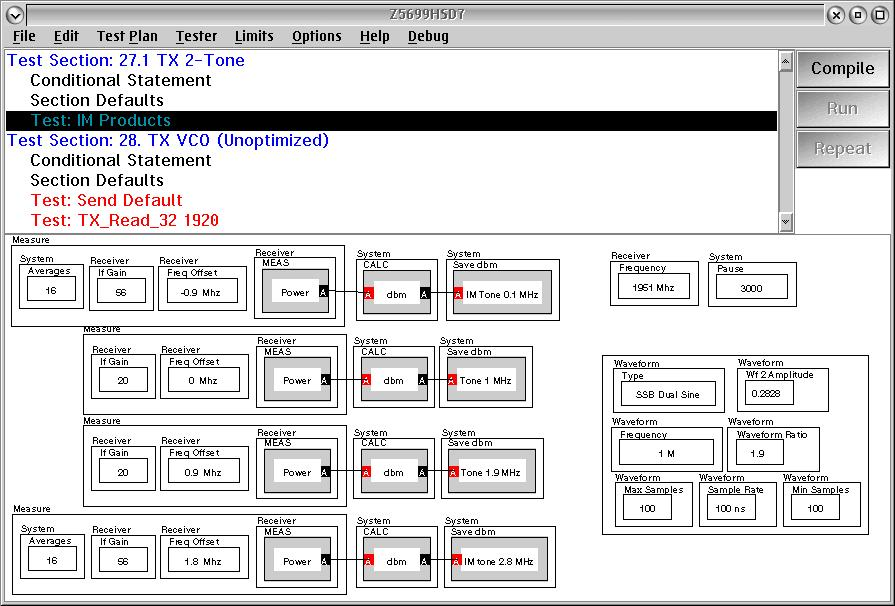One of the modulation types available to the ARBs is SSB Dual Sine. It is an enhancement to the Quad (quadrature) mode that creates two tones at the on the desired side of a single side-band modulator.

The following panel has a measurement example.
It is set up to create a tone at 1 and 1.9 MHz above the suppressed carrier. This would create intermod tones in the DUT 0.1 and 2.8 MHz above the suppressed carrier.

The actual frequencies of measurement are based on the receiver frequency and the receiver frequency offsets.The significant inputs here are the Waveform frequency, Waveform ratio, Max samples, Min sample and sample rate.

The waveform frequency establishes the first tone, The waveform ratio multiplied by the waveform frequency establishes the second tone.

Now the important thing is to create a waveform pattern that is continuous for both frequencies of the two tone. To do that we need to set the max/min and sample rate accordingly. (setting min and maz the same forces the pattern length)

Both frequencies must have a complete an integral number of periods in the arb pattern. So the number of points in the pattern needs to be the least common multiple of the number of points in a single period of each frequency.

As an example, a 1 MHz sine wave will repeat every 1 uSec. The 1.9 MHz sine wave = will repeat in 526.3 nSec...

The least common multiple off the two is 10 uSeconds. (10 X 1 uSec = 19 X 526.3 nSec).

So 10 uSecond pattern is 100 samples at a 100 nSec sample rate.

https://roos.com/docs/MFEY-7HUNW3
ROOS INSTRUMENTS CONFIDENTIAL AND PROPRIETARY